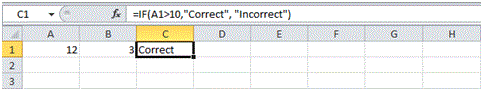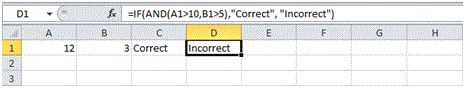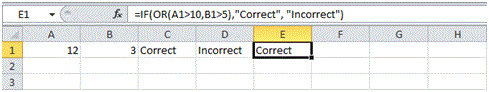# Logical Functions in Excel

## Logical

MS Excel supports logical and conditional functions which enables the user to perform conditional evaluations. This versatility makes MS Excel a must to learn tool in organizations. In this tutorial you will be learning 3 main logical functions that are used for extensive calculations.

IF Function

IF Function is a conditional statement that evaluates a condition and return a customized response for either True or False state. If no custom response is allocated, it will return TRUE or FALSE as default response. You will follow the following steps to learn how to use IF function.

1. Select cell C1 and enter the following function.

= IF(A1>10,”Correct”,”Incorrect”)Now, since the Cell A1 contains value 12 which is greater than 10 therefore, the condition returns true and hence the response shown in the above figure is Correct.

And Function

AND function is a multiplicative nature logical operator that let the user evaluate multiple criteria and return the response TRUE if all given criteria are true otherwise it will return FALSE.

To learn how to use AND operator within IF function, follow the following steps.

1. Select cell D1 and enter the following formula.

=IF(AND(A1>10,B1>5), “Correct”, “Incorrect”)In the above formula, “AND(A1>10,B1>5)” block is used to evaluate two criteria. Here, A1 = 12 which is greater than 10 so this part returns TRUE but B1 = 3 which is not greater than 5 so it returns FALSE, hence, being a multiplicative nature Logical Operator, AND will return FALSE. Therefore, IF statement also returns FALSE and displayed the “Incorrect” response.

Or Function

OR function is an additive nature logical operator that let the user evaluate multiple criteria and return the response TRUE if any given criteria are true otherwise it will return FALSE.

To learn how to use OR operator within IF function, follow the following steps.

1. Select cell E1 and enter the following formula.

=IF(OR (A1>10,B1>5), “Correct”, “Incorrect”)In the above formula, “OR(A1>10,B1>5)” block is used to evaluate two criteria. Here, A1 = 12 which is greater than 10 so this part returns TRUE but B1 = 3 which is not greater than 5 so it returns FALSE, however, being an additive nature Logical Operator, OR will return TRUE. Therefore, IF statement also returns TRUE and displayed the “Correct” response.